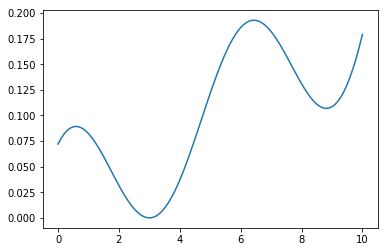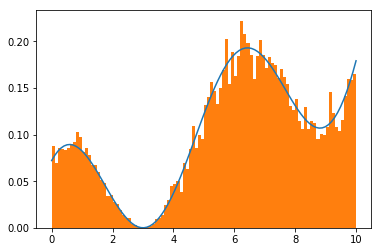MC采样比较经典的是接受-拒绝采样和重要采样，下面直接切入正题....

### 一.接受-拒绝采样¶

（1）如何采样？

（2）$p(x^*)$都是相对大小，如何量化决定去留？

（1）首先，让$q(x)$的概率分布去盖住$p(x)$，即对于任意的$x$都有$q(x)>p(x)$，但是这是不可能的，因为$p(x),q(x)$同为概率分布，必然有$\int_xp(x)=\int_xq(x)=1$，所以如果某个地方有$q(x)>p(x)$，那么必然在其他的地方有$q(x)<p(x)$，所以我们可以对$q(x)$扩大一定的倍数$c>1$使其满足对任意$x$都有$cq(x)>p(x)$，如下图所示，这样我们就解决了上面的第一个问题：如何采样？我们可以按照$q(x)$分布去采样，假如就得到了图中样本点$x^*$，那么接下来就是确定样本去留的问题了（2）$x^*$的去留，可以按照它对应的$\frac{p(x^*)}{cq(x^*)}$来决定，具体可以这样操作，从$U(0,1)$中均匀采样一个$u$，如果$u\leq \frac{p(x^*)}{cq(x^*)}$则保留，否则去掉；

$$q(x^*)\cdot\frac{p(x^*)}{cq(x^*)}=\frac{p(x^*)}{c}\propto p(x^*)$$

### 二.重要采样¶

（1）选择一个容易抽样的分布$q(x)$，从中抽样$x^*$；

（2）设置该样本对应的权重为$\frac{p(x^*)}{q(x^*)}$，称为重要权重，over...

$$q(x^*)\frac{p(x^*)}{q(x^*)}=p(x^*)$$

#### 求期望¶

$$E_{x\sim p(x)}[f(x)]=\int_xp(x)f(x)dx\\ =\int_xf(x)\frac{p(x)}{q(x)}q(x)dx\\ =\int_x[f(x)\frac{p(x)}{q(x)}]q(x)dx\\ =E_{x\sim q(x)}[f(x)\frac{p(x)}{q(x)}]$$

### 三.案例¶

$$p(x)=\frac{1}{1179}[(x-2)^2+(x-5)^3+100cos(x)+106],0<x<10$$

In :
import numpy as np
import matplotlib.pyplot as plt
%matplotlib inline

In :
def func(x):
return ((x-2)*(x-2)+(x-5)*(x-5)*(x-5)+100*np.cos(x)+106)/1179
x=np.linspace(0,10,100)
plt.plot(x,func(x))

Out:
[<matplotlib.lines.Line2D at 0x1ba7fa94e80>]$$q(x)=0.1,0<x<10\\ c=2$$
In :
#采样的样本量
nums=10000
count=0
points=[]
while count<nums:
#首先按照q(x)，随机均匀采样一个样本点
point=np.random.random()*10
#计算p(x)/(c*q(x))
p=func(point)/0.2
#从(0,1)均匀采样一个u
u=np.random.random()
#判断是否保留（即对应了上面的那条线）
if u<p:
points.append(point)
count+=1

In :
#看看效果
plt.plot(x,func(x))
plt.hist(points,normed=True,bins=100)
plt.show()### 四.分析¶

In [ ]: